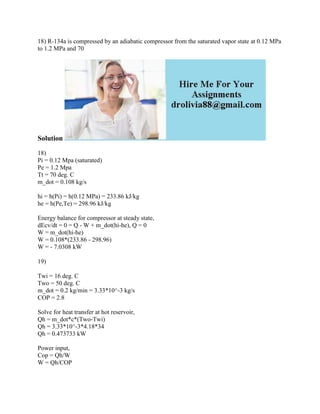Seu SlideShare está sendo baixado. ×

# 18) R-134a is compressed by an adiabatic compressor from the saturated.docx

Anúncio
Anúncio
Anúncio
Anúncio
Anúncio
Anúncio
Anúncio
Anúncio
Anúncio
Anúncio
AnúncioCarregando em…3
×

1 de 2 Anúncio

# 18) R-134a is compressed by an adiabatic compressor from the saturated.docx

18) R-134a is compressed by an adiabatic compressor from the saturated vapor state at 0.12 MPa to 1.2 MPa and 70
Solution
18)
Pi = 0.12 Mpa (saturated)
Pe = 1.2 Mpa
Tt = 70 deg. C
m_dot = 0.108 kg/s

hi = h(Pi) = h(0.12 MPa) = 233.86 kJ/kg
he = h(Pe,Te) = 298.96 kJ/kg

Energy balance for compressor at steady state,
dEcv/dt = 0 = Q - W + m_dot(hi-he), Q = 0
W = m_dot(hi-he)
W = 0.108*(233.86 - 298.96)
W = - 7.0308 kW
19)
Twi = 16 deg. C
Two = 50 deg. C
m_dot = 0.2 kg/min = 3.33*10^-3 kg/s
COP = 2.8

Solve for heat transfer at hot reservoir,
Qh = m_dot*c*(Two-Twi)
Qh = 3.33*10^-3*4.18*34
Qh = 0.473733 kW

Power input,
Cop = Qh/W
W = Qh/COP
W = 0.473733/2.8
W = 0.1692 kW ~ 0.17 kW
20)
COP=Qh/W (for heat pump)
Qc-heat absorbed...not needed in this calculation
Qh-heat rejected Qh= 30000kJ/h
W=3kW
Qh=30 000kJ/h =(25/3) kJ/s =(25/3) kW
COP=(25/3)/(3) = 2.7777....=2.78

u cannot have COP\'s like 0.36 or even 1.18...these values are very low....usually COP\'s range from 2-4.5 where 4.5 is the COP for very efficient heat pumps
.

18) R-134a is compressed by an adiabatic compressor from the saturated vapor state at 0.12 MPa to 1.2 MPa and 70
Solution
18)
Pi = 0.12 Mpa (saturated)
Pe = 1.2 Mpa
Tt = 70 deg. C
m_dot = 0.108 kg/s

hi = h(Pi) = h(0.12 MPa) = 233.86 kJ/kg
he = h(Pe,Te) = 298.96 kJ/kg

Energy balance for compressor at steady state,
dEcv/dt = 0 = Q - W + m_dot(hi-he), Q = 0
W = m_dot(hi-he)
W = 0.108*(233.86 - 298.96)
W = - 7.0308 kW
19)
Twi = 16 deg. C
Two = 50 deg. C
m_dot = 0.2 kg/min = 3.33*10^-3 kg/s
COP = 2.8

Solve for heat transfer at hot reservoir,
Qh = m_dot*c*(Two-Twi)
Qh = 3.33*10^-3*4.18*34
Qh = 0.473733 kW

Power input,
Cop = Qh/W
W = Qh/COP
W = 0.473733/2.8
W = 0.1692 kW ~ 0.17 kW
20)
COP=Qh/W (for heat pump)
Qc-heat absorbed...not needed in this calculation
Qh-heat rejected Qh= 30000kJ/h
W=3kW
Qh=30 000kJ/h =(25/3) kJ/s =(25/3) kW
COP=(25/3)/(3) = 2.7777....=2.78

u cannot have COP\'s like 0.36 or even 1.18...these values are very low....usually COP\'s range from 2-4.5 where 4.5 is the COP for very efficient heat pumps
.

Anúncio
Anúncio

### 18) R-134a is compressed by an adiabatic compressor from the saturated.docx

1. 1. 18) R-134a is compressed by an adiabatic compressor from the saturated vapor state at 0.12 MPa to 1.2 MPa and 70 Solution 18) Pi = 0.12 Mpa (saturated) Pe = 1.2 Mpa Tt = 70 deg. C m_dot = 0.108 kg/s hi = h(Pi) = h(0.12 MPa) = 233.86 kJ/kg he = h(Pe,Te) = 298.96 kJ/kg Energy balance for compressor at steady state, dEcv/dt = 0 = Q - W + m_dot(hi-he), Q = 0 W = m_dot(hi-he) W = 0.108*(233.86 - 298.96) W = - 7.0308 kW 19) Twi = 16 deg. C Two = 50 deg. C m_dot = 0.2 kg/min = 3.33*10^-3 kg/s COP = 2.8 Solve for heat transfer at hot reservoir, Qh = m_dot*c*(Two-Twi) Qh = 3.33*10^-3*4.18*34 Qh = 0.473733 kW Power input, Cop = Qh/W W = Qh/COP
2. 2. W = 0.473733/2.8 W = 0.1692 kW ~ 0.17 kW 20) COP=Qh/W (for heat pump) Qc-heat absorbed...not needed in this calculation Qh-heat rejected Qh= 30000kJ/h W=3kW Qh=30 000kJ/h =(25/3) kJ/s =(25/3) kW COP=(25/3)/(3) = 2.7777....=2.78 u cannot have COP's like 0.36 or even 1.18...these values are very low....usually COP's range from 2-4.5 where 4.5 is the COP for very efficient heat pumps